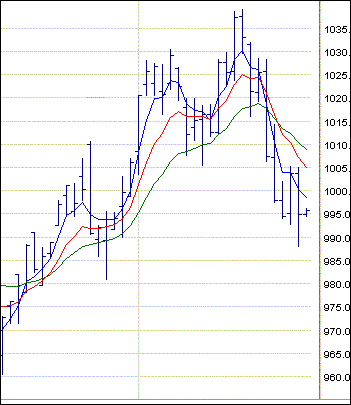This indicator is a variation of the more basic simple moving average indicator. It lends more weight to recent data than the simple moving average, which actually drops old data from its sample. In this case the historical data is always carried forward by using the result from the prior period as a basis for the calculation.

EMA = EMAp + {K * (Price - EMAp)}

EMA = exponential moving average
EMAp = the previous period exponential moving average
K = smoothing constant
Price = current price

K the smoothing constant is derived from the time period selected by the user according to the following formula

K = 2/n+1

Where n is the period chosen by the user

In the example below the shortest period is represented by the blue line (n = 4), the next is the red line (n = 9) and the longest period is the green line (n = 14). These parameters (4, 9, 14) can be selected by the user and in this example appear overlayed on a daily chart.### How to use the indicator

A trading signal is issued when a short term average line crosses over a longer term average line. A buy signal is produced when the short term average crosses above the longer tem average. A sell signal is produce when the short term average line crosses below the long term average line.

This indicator is slightly more sensitive than the simple moving average indicator, but despite this the smoothing constant can actually reduce the amount of whipsaw you would expect from this heightened sensitivity.

In the above example, a buy signal was initially triggered when the blue, short term, average line crossed above the red, medium term, average line and again as it crossed above the green, long term, average line. Yet another final or confirmation signal was generated for buyers as the red line crossed above the green average line.

An exit signal and/or fresh sell signal was generated toward the end of the chart when the blue line first crossed below the red line and again when it crossed the green line. The final sell signal was produced when the red line crossed below the green line.

There are other ways to use moving average indicators and these will be covered in the appropriate section on simple moving averages.

Disclaimer
© The MacLean Group Pty Ltd ACN 096 967 038. All rights reserved 2003. This article has been prepared by The MacLean Group and licensed to ASX. The views are those of the author and not of ASX. This material is educational and it is not intended to constitute financial advice.